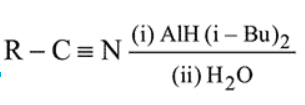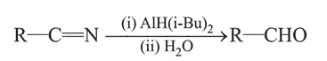# The major product of following reaction is:

Question:

The major product of following reaction is:1. $\mathrm{RCOOH}$

2. $\mathrm{RCONH}_{2}$

3. $\mathrm{RCHO}$

4. $\mathrm{RCH}_{2} \mathrm{NH}_{2}$

Correct Option: , 3

Solution:The reduction of nitriles to aldehydes can be done using DIBAL-H[AIH(i-Bu) $_{2}$ ].